# Test: Introduction To 3D Geometry

## 20 Questions MCQ Test Mathematics (Maths) Class 12 | Test: Introduction To 3D Geometry

Description
This mock test of Test: Introduction To 3D Geometry for JEE helps you for every JEE entrance exam. This contains 20 Multiple Choice Questions for JEE Test: Introduction To 3D Geometry (mcq) to study with solutions a complete question bank. The solved questions answers in this Test: Introduction To 3D Geometry quiz give you a good mix of easy questions and tough questions. JEE students definitely take this Test: Introduction To 3D Geometry exercise for a better result in the exam. You can find other Test: Introduction To 3D Geometry extra questions, long questions & short questions for JEE on EduRev as well by searching above.
QUESTION: 1

### Find the direction cosines of a line which makes equal angles with all three the coordinate axes.​

Solution: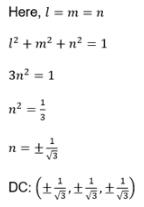QUESTION: 2

### Three planes, viz the XY Plane, XZ Plane and the YZ Plane divide the space into eight parts. Each part is called an OCTANT. What is the relation between these three planes​

Solution:

The three mutually perpendicular coordinate planes which in turn divide the space into eight parts and each part is known as octant.

QUESTION: 3

### The co-ordinates of the vertices of the triangle are A(-2, 3, 6), B(-4, 4, 9) and C(0, 5, 8). The direction cosines of the median BE are:​

Solution: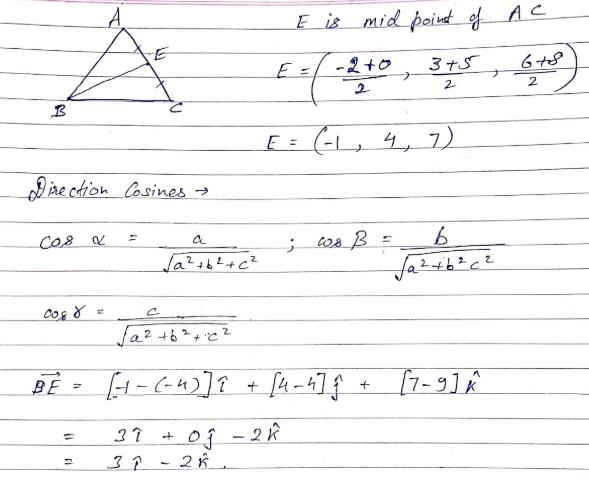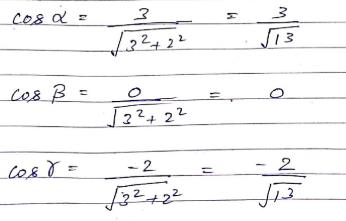QUESTION: 4

If the direction cosines of a line from the positive X-axis and Y-axis are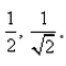The angle of the line through Z-axis is:​

Solution: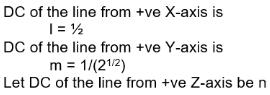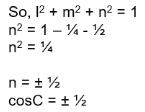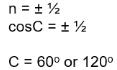QUESTION: 5

The direction cosines of the line equally inclined with the axes are:​

Solution: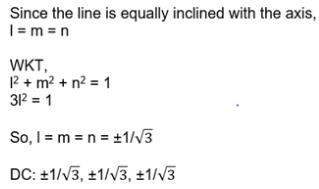QUESTION: 6

The direction cosines of the line whose direction ratios are 6, – 6, 3 are:

Solution: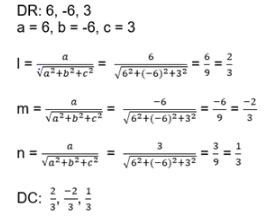QUESTION: 7

Find the direction cosines of the x axis.​

Solution:

To find Direction Cosines of X-axis.
Take any two points on X-axis : A(a,0,0) & B(b,0,0)
DR of AB : (b-a,0,0)
DC of AB : ((b-a)/(((b-a)2 + 0 + 0)1/2), 0, 0)
: ((b-a)/(b-a) , 0 , 0)
: (1,0,0)

QUESTION: 8

The direction cosines of the line joining the points (2, -1, 8) and (-4, -3, 5) are:

Solution:

Pt. A(2, -1, 8)
Pt. B(-4, -3, 5)
Direction Ratio DR of AB : ( -4-2 , -3+1 , 5-8 )
: (-6,-2,-3)
Direction cosine of AB : ( -6/(62+22+32)1/2 , -2/(62+22+32)1/2 , -3/(62+22+32)1/2)
: ( -6/7, -2/7, -3/7)

QUESTION: 9

What are direction numbers of a line.​

Solution:

The numbers which are proportional to direction cosines of a line are called direction numbers of the line.

QUESTION: 10

What are direction ratios of a line.​

Solution:
QUESTION: 11

Find the direction cosines of the side AB of the triangle whose vertices are A(3, 5, -4), B(-1, 1, 2) and C(-5, -5, -2)

Solution: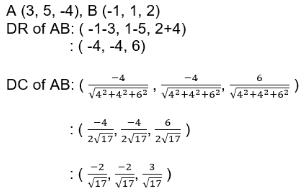QUESTION: 12

If l, m , n are the direction cosines of any line, then sum of the squares of the direction cosines of the line is always​

Solution:
QUESTION: 13

If a line has the direction ratios -4, 18, -12 then what are its direction cosines?​

Solution:

DR of the line :  (-4, 18 -12)
DC of the line : (-4/k, 18/k, -12/k)
where k = ((42) + (182) + (12)2)1/2
= (16 + 324 + 144)1/2
= (484)1/2
= 22
So, DC : (-4/22, 18/22, -12/22)
: (-2/11 , 9/11 , -6/11)

QUESTION: 14

The direction cosines of the line equally inclined with the axes, are:​

Solution: Cos^2 alpha+cos ^2 beta +cos^ 2 gamma =1
put alpha =beta. =gamma
we get cosalpha= 1/√3
DC's of given line (1/√3,1/√3,1/3)
QUESTION: 15

If a line makes angles 45°,150°, 135°, with x, y and z-axes respectively, find its direction cosines.

Solution: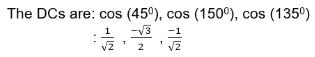QUESTION: 16

Find the equation of the set of points which are equidistant from the points (1, 2 , 3) and (3, 2, -1)​

Solution:

Pt. A(1, 2 , 3)
Pt. B(3, 2, -1)
Let P(x,y,z)
So, AP = BP
((x-1)2 + (y-2)2 + (z-3)2)1/2 = ((x-3)2 + (y-2)2 + (z+1)2)1/2
(x-1)2 + (y-2)2 + (z-3)2) = (x-3)2 + (y-2)2 + (z+1)2
x2 +1 -2x + y2 + 4 - 4y + z2 + 9 – 6z = x2 +9 -6x + y2 + 4 - 4y + z2 + 1 + 2z
4x – 8z = 0
x – 2z = 0

QUESTION: 17

If a line in the ZX-plane makes an angle 60o with Z-axis, the direction cosines of this line are:

Solution: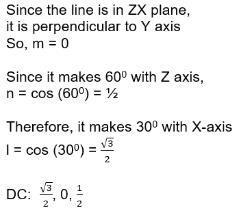QUESTION: 18

A line makes angles α, β, γ with the positive directions of X-axis, Y-axis and Z-axis, respectively, then the directions cosines of the line are:

Solution:

cos α, cos β, cos γ
By the definition of Direction Cosines

QUESTION: 19

The signs of the X,Y and Z coordinates of a point that lies in the octant OXYZ’ is​

Solution:

X,Y,Z imply positive X,Y,Z axis & X’,Y’,Z’ imply negative X,Y,Z axis.
So, OXYZ’ will have a point of signs (+, +, -).

QUESTION: 20

If a line in the ZX-plane makes an angle 30o with Z-axis, the direction cosines of this line are:

Solution: The line makes 30o with z-axis

Since it is in z-x axis the angle made with y-axis is 90

And angle made by x-axis is 60

Therefore direction cosines are

1/2, 0, √3/2